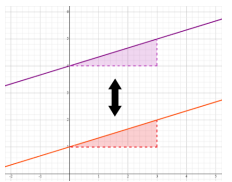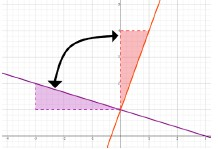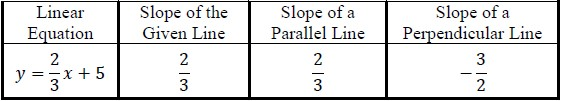# MA.912.AR.2.3Export Print
Write a linear two-variable equation for a line that is parallel or perpendicular to a given line and goes through a given point.

### Clarifications

Clarification 1: Instruction focuses on recognizing that perpendicular lines have slopes that when multiplied result in -1 and that parallel lines have slopes that are the same.

Clarification 2: Instruction includes representing a line with a pair of points on the coordinate plane or with an equation.

Clarification 3: Problems include cases where one variable has a coefficient of zero.

General Information
Subject Area: Mathematics (B.E.S.T.)
Strand: Algebraic Reasoning
Status: State Board Approved

## Benchmark Instructional Guide

### Terms from the K-12 Glossary

• Linear Equation
• Rotation
• Slope
• Translation

### Vertical Alignment

Previous Benchmarks

Next Benchmarks

### Purpose and Instructional Strategies

In grade 8, students determined whether a graphed system of linear equations resulted in one solution, no solution (parallel lines) or infinitely many solutions. In Algebra I, students write linear two-variable equations that are parallel or perpendicular to one another. In Geometry, students will use slope criteria of parallel and perpendicular lines to justify postulates, relationships or theorems.
• Instruction includes allowing students to explore the transformations of two lines using graphing software or other technology. If students don’t have their own computers, use your own and let students direct the exploration.
• For example, using graphing software, ask students to use the sliders to discover two lines that are parallel. Once students achieve this, write the equations of these two lines on the board and ask them to find two different lines that are parallel. Write the equations for these lines on the board. Repeat this for multiple pairs of lines. As students explore, ask them to find patterns in the equations they develop (MTR.5.1). Guide their discussion to focus on the fact that parallel lines have equivalent slopes (MTR.4.1).
• Repeat this exercise for perpendicular lines.
• When students establish connections and understanding of slopes of parallel and perpendicular lines, tie them back to their work with transformations in Grade 8 (MTR.5.1).
• For example, parallel lines can be translated to coincide with each other without changing slope.• For example, perpendicular lines can be rotated 90° about the point of intersection to coincide with each other. Use slope to draw congruent triangles on each line and show the change in slope to an opposite reciprocal after rotation.• Once students understand slope relationships, instruction should guide them to utilize slope and a point on the line to develop the point-slope equation for the line.
• Students can also develop slope-intercept equations for the line. Students should have prior knowledge from their work in MA.912.AR.2.2 that $x$ and $y$ in a linear equation represent points on the corresponding line. Direct students to see that substituting the slope of a line ($m$) and the coordinates of a point on that line ($x$, $y$) into slope-intercept form ($y$ = $m$$x$ + $b$) allows them to solve to find the $y$-intercept.

### Common Misconceptions or Errors

• Some students may forget to change the sign of the slope when working through perpendicular line problems. Others may change the sign correctly but forget to make the slope a reciprocal. In both cases, consider having the student sketch a rough graph or use graphing software to check their result.

### Strategies to Support Tiered Instruction

• Instruction provides opportunities to identify parallel or perpendicular lines using a graphing tool, graphing software or sketching a rough graph.
• Instruction includes opportunities to graph a linear function on a coordinate grid. Teacher models using a piece of spaghetti or other straight item (such as a pencil) to show a line parallel or perpendicular to the one they graphed and estimate the slope of the straight item. Students can compare that estimate with the slope of the parallel or perpendicular line they determined algebraically. 1
• For example, if the given line has a slope of −$\frac{\text{1}}{\text{2}}$ and the student determines incorrectly that the perpendicular slope is −2, they can visually see the mistake when using a straight object that is placed perpendicular to the graph of the given line.
• Teacher provides opportunities to practice identifying the slope of parallel and perpendicular lines using a graphic organizer. To follow up this activity, have students do a card match where they match a linear equation to the slope of a parallel line and the slope of a perpendicular line.
• For example, the organizer below could be used to identify the slope of the parallel and perpendicular line of the given equation.• Write a linear function for a line that is parallel to $f$($x$) = $\frac{\text{1}}{\text{3}}$$x$ − 8 and passes through the 3 point (−7, 0).

• Part A. A vertical line that passes through the point (−0.42, 7.8) is perpendicular to another line. What could be the equation of the second line?
• Part B. Graph both lines on a coordinate plane. What do you notice about the lines?
• Part C. Compare your graph with a partner

### Instructional Items

Instructional Item 1
• Write a linear function for a line that passes through the point (4, –3) and is perpendicular to $y$ = 0.25$x$ + 9.

Instructional Item 2
• Write an equation for the line that passes through (3, 14) and is parallel to the line that passes through (10, 2) and (25, 15).

*The strategies, tasks and items included in the B1G-M are examples and should not be considered comprehensive.

## Related Courses

This benchmark is part of these courses.
1200310: Algebra 1 (Specifically in versions: 2014 - 2015, 2015 - 2022, 2022 and beyond (current))
1200320: Algebra 1 Honors (Specifically in versions: 2014 - 2015, 2015 - 2022, 2022 and beyond (current))
1200370: Algebra 1-A (Specifically in versions: 2014 - 2015, 2015 - 2022, 2022 and beyond (current))
1200400: Foundational Skills in Mathematics 9-12 (Specifically in versions: 2014 - 2015, 2015 - 2022, 2022 and beyond (current))
7912080: Access Algebra 1A (Specifically in versions: 2014 - 2015, 2015 - 2018, 2018 - 2019, 2019 - 2022, 2022 and beyond (current))
1200315: Algebra 1 for Credit Recovery (Specifically in versions: 2014 - 2015, 2015 - 2022, 2022 and beyond (current))
1200375: Algebra 1-A for Credit Recovery (Specifically in versions: 2014 - 2015, 2015 - 2022, 2022 and beyond (current))
7912075: Access Algebra 1 (Specifically in versions: 2014 - 2015, 2015 - 2018, 2018 - 2019, 2019 - 2022, 2022 and beyond (current))

## Related Access Points

Alternate version of this benchmark for students with significant cognitive disabilities.
MA.912.AR.2.AP.3: Select a linear two-variable equation in slope intercept form for a line that is parallel or perpendicular to a given line and goes through a given point.

## Related Resources

Vetted resources educators can use to teach the concepts and skills in this benchmark.

## Formative Assessments

Writing Equations for Parallel Lines:

Students are asked to identify the slope of a line parallel to a given line and write an equation for the line given a point.

Type: Formative Assessment

Writing Equations for Perpendicular Lines:

Students are asked to identify the slope of a line perpendicular to a given line and write an equation for the line given a point.

Type: Formative Assessment

## Lesson Plans

Graphing vs. Substitution. Which would you choose?:

Students will solve multiple systems of equations using two methods: graphing and substitution. This will help students to make a connection between the two methods and realize that they will indeed get the same solution graphically and algebraically.  Students will compare the two methods and think about ways to decide which method to use for a particular problem. This lesson connects prior instruction on solving systems of equations graphically with using algebraic methods to solve systems of equations.

Type: Lesson Plan

Investigating Lines With Our Minds!:

A discovery investigation for the slopes of parallel and perpendicular lines. Students write the equations of lines parallel and/or perpendicular to a given line through a given point. Directions for using graph paper or x-y coordinate pegboards are given.

Type: Lesson Plan

## MFAS Formative Assessments

Writing Equations for Parallel Lines:

Students are asked to identify the slope of a line parallel to a given line and write an equation for the line given a point.

Writing Equations for Perpendicular Lines:

Students are asked to identify the slope of a line perpendicular to a given line and write an equation for the line given a point.

## Student Resources

Vetted resources students can use to learn the concepts and skills in this benchmark.

## Parent Resources

Vetted resources caregivers can use to help students learn the concepts and skills in this benchmark.